# ISOWEEKNUM Function

Get the ISO week number for a given date

## What is the ISOWEEKNUM Function?

The ISOWEEKNUM Function in Excel is a Date & Time Function used for finding out the ISO week number of the year for the given date value (e.g., week number 37 of 52 weeks in a year). The function was introduced in MS Excel 2013.

### Formula

=ISOWEEKNUM(date)

Where date is a required argument. Excel stores dates in a serial date format. It represents the days from January 1, 1900. So January 1, 1900 would be the serial number 1 and January 1, 2009 would be 39814, as it is 39814 days after January 1, 1900.

This function will return a number between 1 to 52, specifying in which week the date falls.

### How to use ISOWEEKNUM to get week number in Excel?

To understand the uses of this function, let’s consider a few examples.

#### Example 1

Let’s assume the date today is April 1, 2017. If we wish to find out which week of the year it is, we can use the ISOWEEKNUM function in this manner: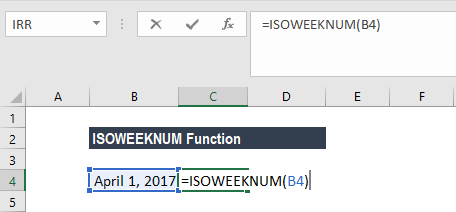Column B displays the date and Column C the result of the function.

#### Example 2

For the ISOWEEKNUM function, the week starts on Monday. As January 1 was a Sunday in 2017, when you use this function, it will show 52 as the result: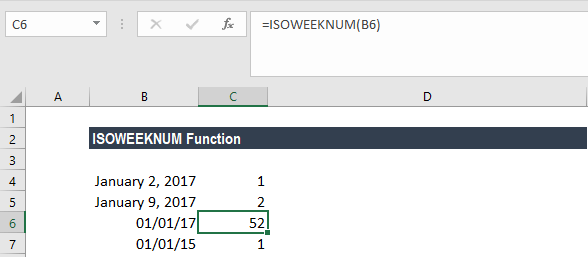So, the first week of the year will start from January 2, 2017 and end on January 8, 2017. As shown below, and dates between January 2, 2017 to January 8, 2017 will be the first week and then January 9 will come under the second week.

Now let’s assume the date is January 1, 2015, which is a Thursday. The formula would take that as week 1 of the year as shown above.

#### Example 3

Let’s see how this formula can be used along with another Date & Time function. Let’s assume I wish to find out the date today and the current week of the year. I can use the formula =ISOWEEKNUM (TODAY ()) as shown below: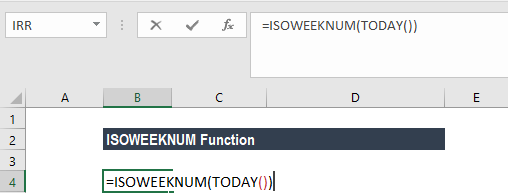As today is March 6, 2018, ISOWEEKNUM will return 10 as the result: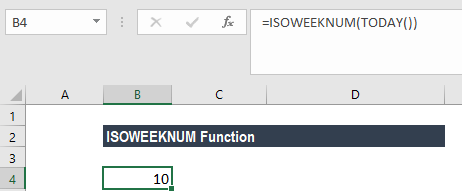Further, we then need to find out how many weeks are left from the last week, that is the 52nd week. In this scenario, we can modify the formula as:

=52 -ISOWEEKNUM(TODAY())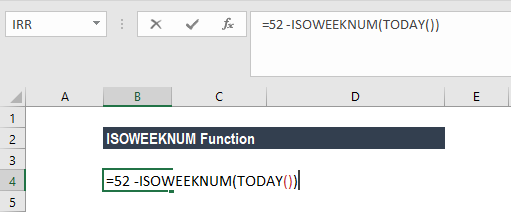We get the result below: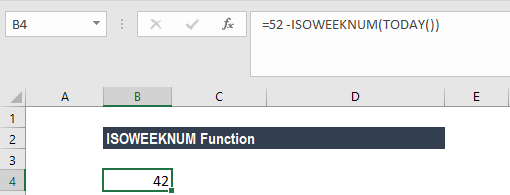### A few pointers for the ISOWEEKNUM Function

1. #NUM! error – Occurs when the date argument given is not a valid number.
2. #VALUE! error – Occurs when the date argument given is not a valid date type.
3. The ISOWEEKNUM function returns the week number on the basis of European ISO standard ISO8601:2000.
4. As we are aware that MS Excel stores dates sequentially in numbers, the function can be used even when there’s a serial number given. For example, if we are given serial number 39,814 then ISOWEEKNUM will return the result as 1.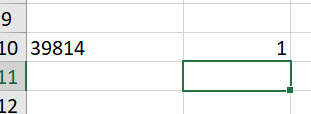1. If we are using an older version of MS Excel, we can use the function WEEKNUM. By default, the WEEKNUM function uses an arrangement where Week 1 begins on January 1, and Week 2 begins on the next Sunday (when the return_type argument is omitted, or supplied as 1).

However, with MS Excel 2010 for Windows and MS Excel 2011 for Mac, we can generate an ISO week number using 21 as the return_type: =WEEKNUM(date,21).

1. There is no built-in worksheet function for ISO weeks before MS Excel 2010. Hence, if we are using data from different Excel versions, it would be good to use the function ISOWEEKNUM.

Thanks for reading CFI’s guide to important Excel functions! By taking the time to learn and master these functions, you’ll significantly speed up your financial analysis. To learn more, check out these additional CFI resources:

• Excel Functions for Finance